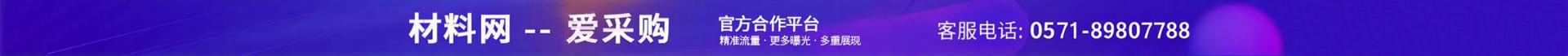•••e="63" et="1">

me="68539">

e="5978" et="2">

e="2514" et="3">

e="3290" et="5">